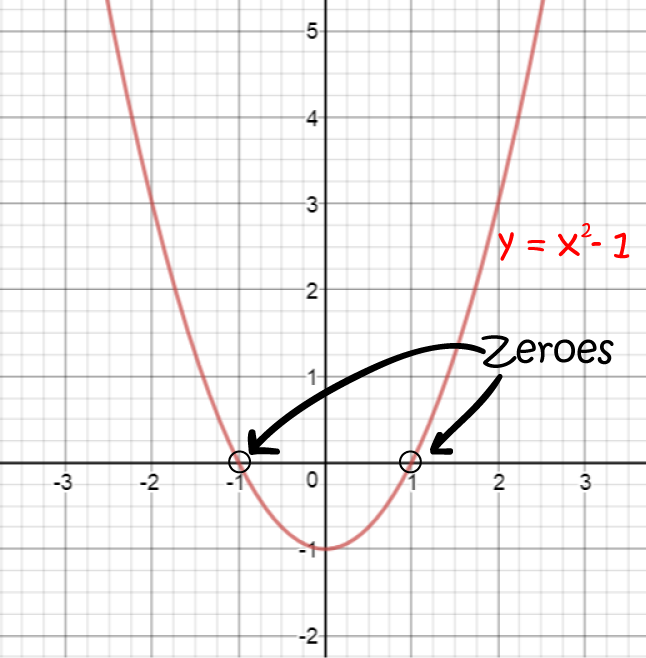# Definition of Zero (of a function)A zero of a function $f(x)$ is a place where $f(x) = 0$. In other words, it's a place where the graph of $f(x)$ crosses the $x$-axis.

The function in the picture is $f(x) = x^2 -1$. It crosses the $x$-axis at $x = 1$ and $x = -1$. So, it has zeroes at $x = 1$ and $x = -1$.

Many people call the zeroes of a function the "roots" of a function. They mean exactly the same thing.

### Description

The aim of this dictionary is to provide definitions to common mathematical terms. Students learn a new math skill every week at school, sometimes just before they start a new skill, if they want to look at what a specific term means, this is where this dictionary will become handy and a go-to guide for a student.

### Audience

Year 1 to Year 12 students

### Learning Objectives

Learn common math terms starting with letter Z

Author: Subject Coach
You must be logged in as Student to ask a Question.